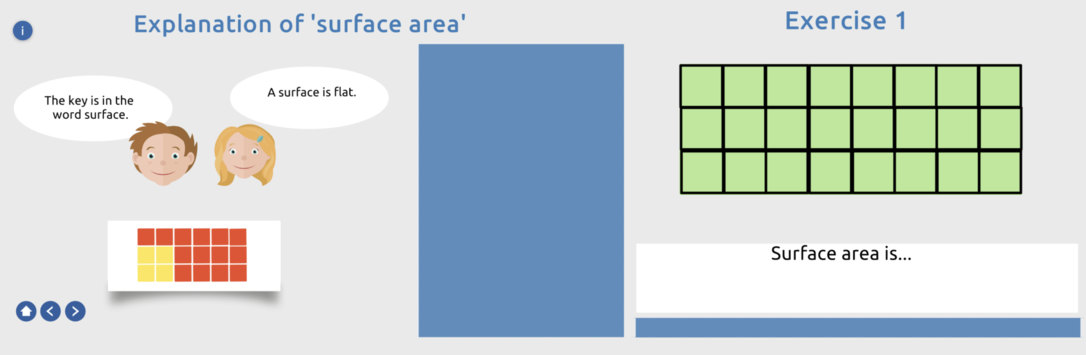# Calculating area

## Key Stage 2

View this page on a larger screen to send this lesson directly to Presenter

### Purpose

Calculate area using squares
Calculate area using the formula: length x width
Calculating the area of square, rectangle and triangle

Start calculating the area of different shapes.
Teach the students what the surface area of a shape is and how to calculate it.
Use the formula for calculating the area, namely length x width.
Students can complete calculating the area using different exercises.